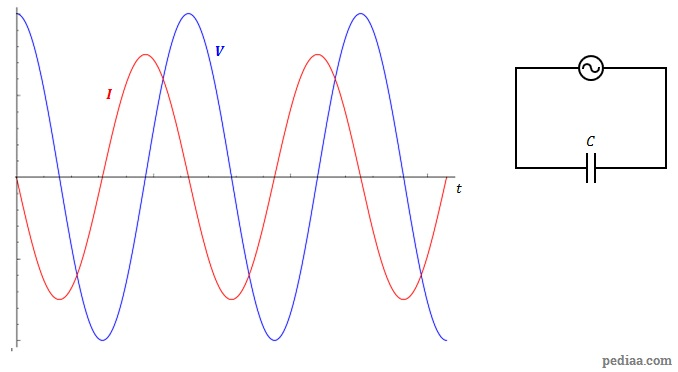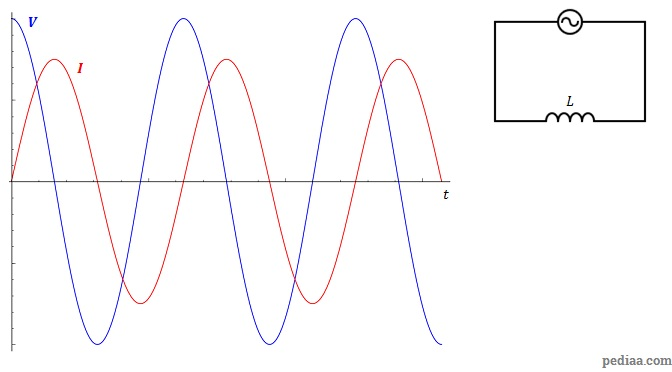# Difference Between Resistance and Reactance

## Main Difference – Resistance vs. Reactance

Resistance and Reactance are properties of an electrical circuit that opposes current. The main difference between resistance and reactance is that resistance measures the opposition to a flow of current, whereas reactance measures the opposition to a change in current.

## What is Resistance

The resistance ($R$) of a component in a circuit is the potential difference ($V$) across the component divided by the current ($I$) through the component:$R=\frac{V}{I}$

If a resistor is added to a circuit powered by an alternating current, the changes in current will occur simultaneously with changes in voltage.Current and voltage across a resistor in an AC circuit changes at the same phase.

## What is Reactance

Reactance is an opposition to a change in current. Reactance is a property of capacitors or inductors because their functionality is intimately related to the rates of change of current and voltage.

## Capacitive Reactance

When a capacitor is connected to a circuit with an alternating power supply, the voltage across the capacitor and the current through the capacitor does not change simultaneously. The potential difference across the capacitor is changing in response to the alternating power supply. The rate at which charges accumulate/leave the plates is greater when the potential difference is changing at a faster rate. Consequently, the current is at a maximum when the  potential difference is around 0 (this is when the rate of change of potential difference is highest). The graph below illustrates how the current and potential difference changes. We say that the current leads potential difference by a quarter-cycle (the maximum voltage comes quarter-a-cycle after the current):Capacitive reactance: for a capacitor, the maximum current is ahead of the maximum voltage by a quarter-cycle.

The maximum potential difference across the capacitor,$V_C$ is given in terms of the maximum current$I$ in the circuit and the capacitive reactance of the capacitor,$X_C$, as$V_C=IX_C$. For a capacitor with capacitance$C$ connected to a circuit whose alternating current has a frequency$f$, the capacitive reactance is given by:$X_C=\frac{1}{2\pi fC}$

## Inductive Reactance

When the current across an inductor changes, a potential difference develops across it. The potential difference is proportional to the rate of change of current. Consequently, for inductors potential difference leads the current by a quarter cycle.Inductive reactance: for an inductor, the maximum voltage is ahead of the maximum current by a quarter-cycle.

The potential difference across the inductor$V_L$ is given gy$V_L=IX_L$, where$I$ is the maximum current and$X_L$ is the inductive reactance. If the inductance of the inductor is$L$ and the frequency of the alternating current is$f$, then:$X_L=2\pi fL$

Note that capacitive and inductive reactance can both be described as a ratio of potential difference to current. As a result, both types of reactances have units of ohms (Ω).

## Difference Between Resistance and Reactance

### Variation of Current

Resistance is a property of a component that opposes a flow of current.

Reactance is a property of a component that opposes a change in current.

### Power Dissipation

Resistance leads to a dissipation of power.

Reactance does not lead to power dissipation (for ideal capacitors and inductors).